﻿ 基于卷积神经网络的手写体深度学习算法研究 Research on Deep Learning Algorithm about Handwriting Based on the Convolutional Neural Network

Computer Science and Application
Vol. 08  No. 11 ( 2018 ), Article ID: 27720 , 9 pages
10.12677/CSA.2018.811196

Research on Deep Learning Algorithm about Handwriting Based on the Convolutional Neural Network

Liying Ma

Shanghai DianJi University, Shanghai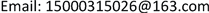Received: Nov. 7th, 2018; accepted: Nov. 19th, 2018; published: Nov. 26th, 2018ABSTRACT

Deep learning is a collection of algorithms that are used to solve related problems such as image and text. As an important algorithm for deep learning, convolutional neural network is especially good at image processing field. The convolution neural network extracts the various features of the image through the convolution kernel, and its orders of magnitude are greatly reduced by weight sharing and pooling. In this paper, MINST handwritten database is used as training sample to discuss the reverse propagation mechanism of weight value of convolutional neural network, and the implementation method with MATLAB; In order to obtain the optimal correction parameters and learning rate, the problems of gradient disappearance of activation functions tanh and relu were analyzed and optimized, and the improved activation function was trained.

Keywords:Convolutional Neural Network, Deep Learning, Handwriting Recognition1. 引言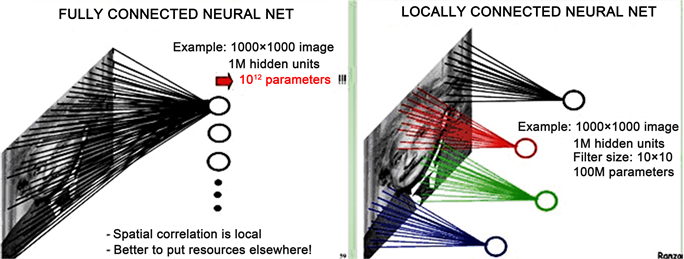Figure 1. Local perception schematic of convolutional neural network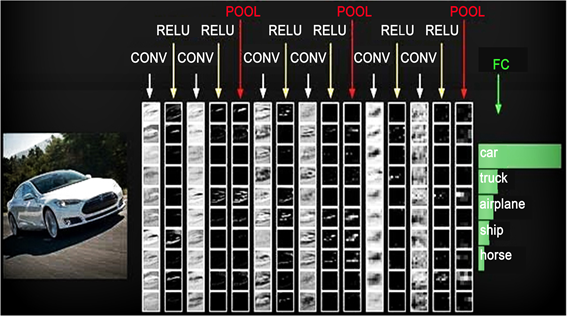Figure 2. Calculation schematic of convolutional neural network

2. 卷积神经网络的卷积核传递

$C=\frac{1}{2}{\sum }_{j}{\left({y}_{j}-{a}_{j}\right)}^{2}$ (1)

${y}_{j}$ 为输出层第j个神经元的实际值， ${a}_{j}$ 为第j个神经元的理想值，采用方程(1)的形式定义目标函数，方便后面的求导计算：

$\frac{\partial C}{\partial {a}_{j}}=\left({a}_{j}-{y}_{j}\right)$ (2)

${\delta }_{j}^{L}=\frac{\partial C}{\partial {z}_{j}^{L}}=\frac{\partial C}{\partial {a}_{j}^{L}}\frac{\partial {a}_{j}^{L}}{\partial {z}_{j}^{L}}$ (3)

${z}_{j}^{L}$ 代表第L层，即输出层第j个神经元， ${a}_{j}^{L}$ 为神经元 ${z}_{j}^{L}$ 通过激活函数 $f\left(z\right)$ 后的激活值，那么即可求出输出层第j个神经元的误差为：

${\delta }_{j}^{L}=\left({a}_{j}-{y}_{j}\right){f}^{\prime }\left(z\right)$ (4)

${\delta }_{j}^{l}=\frac{\partial C}{\partial {z}_{j}^{l}}=\underset{k}{\sum }\frac{\partial C}{\partial {z}_{k}^{l+1}}\frac{\partial {z}_{k}^{l+1}}{\partial {z}_{j}^{l}}=\underset{k}{\sum }\frac{\partial {z}_{k}^{l+1}}{\partial {z}_{j}^{l}}{\delta }_{k}^{l+1}$ (5)

${z}_{k}^{l+1}=\underset{j}{\sum }{\omega }_{kj}^{l+1}{a}_{j}^{l}+{b}_{k}^{l+1}=\underset{j}{\sum }{\omega }_{kj}^{l+1}f\left({z}_{j}^{l}\right)+{b}_{k}^{l+1}$ (6)

$\frac{\partial {z}_{k}^{l+1}}{\partial {z}_{j}^{l}}={w}_{kj}^{l+1}{f}^{\prime }\left({z}_{j}^{l}\right)$ (7)

${\delta }_{j}^{l}=\underset{k}{\sum }{\omega }_{kj}^{l+1}{\delta }_{k}^{l+1}{f}^{\prime }\left({z}_{j}^{l}\right)$ (8)

$\frac{\partial C}{\partial {\omega }^{l}}=\frac{\partial C}{\partial {z}^{l}}\frac{\partial {z}^{l}}{\partial {\omega }^{l}}={\delta }^{l}{\left({a}^{l-1}\right)}^{T}$ (9)

$\frac{\partial C}{\partial {b}^{l}}=\frac{\partial C}{\partial {z}^{l}}\frac{\partial {z}^{l}}{\partial {b}^{l}}={\delta }^{l}$ (10)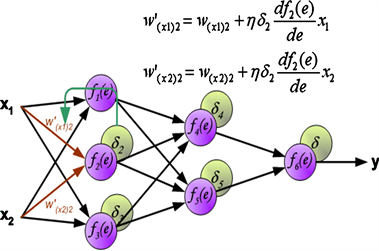Figure 3. The update process of the neural network weight

${\delta }_{j}^{l}=upsample\left({\delta }_{j}^{l+1}\right)\otimes {f}^{\prime }\left({z}_{j}^{l+1}\right)$ (11)

for j = 1 : numel(net.layers{l}.a)

net.layers{l}.d{j} = net.layers{l}.a{j} .* (1 - net.layers{l}.a{j}) .* (expand(net.layers{l + 1}.d{j}, [net.layers{l + 1}.scale net.layers{l + 1}.scale 1]) / net.layers{l + 1}.scale ^ 2);

end

net.layers{l}.a为第1层神经元，numel(net.layers{l}.a为卷积层神经元的个数，这里net.layers{l}.a{j} .* (1 - net.layers{l}.a{j})是对激活函数f(z)求导。expand(net.layers{l + 1}.d{j}, [net.layers{l + 1}.scale net.layers{l + 1}.scale 1]) / net.layers{l + 1}.scale ^ 2是上采样操作。

${\delta }_{j}^{l}=\underset{k}{\sum }\left({\delta }_{k}^{l+1}\otimes rot180\left({\omega }_{jk}\right)\right)$ (12)

for i = 1 : numel(net.layers{l}.a)

z= zeros(size(net.layers{l}.a{1}));

for j = 1 : numel(net.layers{l + 1}.a)

z = z + convn(net.layers{l + 1}.d{j}, rot180(net.layers{l + 1}.k{i}{j}), 'full');

end

net.layers{l}.d{i} = z;

end

numel(net.layers{l}.a)为池化层神经元的个数，numel(net.layers{l + 1}.a)为池化层下一层即卷积层神经元的个数，net.layers{l}.d{i}为池化层第1层第i个神经元的误差。

$\frac{\partial C}{\partial {\omega }_{jk}^{l}}=\frac{\partial C}{\partial {z}_{j}^{l}}\frac{\partial {z}_{j}^{l}}{\partial {\omega }_{jk}^{l}}={\delta }^{l}*rot\left({a}_{k}^{l-1}\right)$ (13)

$\frac{\partial C}{\partial {b}_{j}^{l}}=\sum {\delta }_{j}^{l}/size\left({\delta }_{j}^{l}\right)$ (14)

for j = 1 : numel(net.layers{l}.a)

for i = 1 : numel(net.layers{l - 1}.a)

net.layers{l}.dk{i}{j}=convn(flipall(net.layers{l-1}.a{i}),net.layers{l}.d{j},'valid')/size(net.layers{l}.d{j}, 3);

net.layers{l}.db{j} = sum(net.layers{l}.d{j}(:)) / size(net.layers{l}.d{j}, 3);

end

numel(net.layers{l}.a 为卷积层l神经元的个数，net.layers{l}.dk{i}{j}为第l层卷积核k{i}{j}的误差。net.layers{l}.db{j}为卷积层偏置的误差。

3. 深度学习算法优化

$f\left(x\right)=\frac{2}{1+{\text{e}}^{-2x}}-1$ (15)Figure 4. Primitive function and derived function of the tanh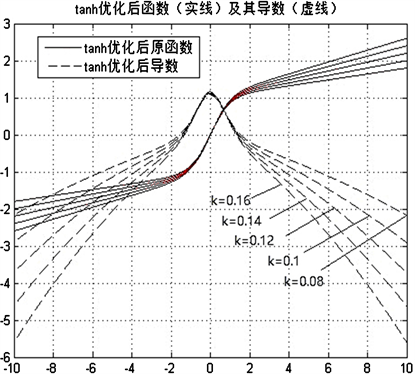Figure 5. Primitive function and derived function of the optimized tanh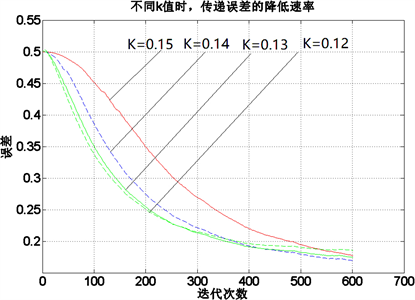Figure 6. The influence of different k values on the calculation rate of error function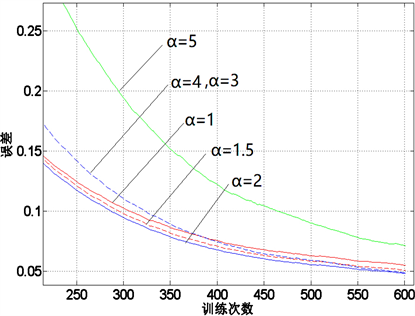Figure 7. Error reduction in different learning rates

Tanh激活函数在求导计算时，涉及指数运算，在处理大规模数据输入时，时间代价较大，为了更加快速地进行误差传递，采用 $f\left(x\right)=\mathrm{max}\left(0,x\right)$ 即Relu函数激活   ，该激活函数及其导数的图像如图8所示。从图中可以看出，当输入大于0时，其梯度快速增加，解决梯度消失问题，提供神经网络稀疏表达能力，但是当输入小于0时，梯度为0，在训练过程中权值无法更新，造成部分神经元死亡。为了解决这一问题，需要对max(0, x)激活函数进行改进。为了避免梯度消失的情况出现，将激活函数小于0范围内，增加一个修正系数α，即： $f\left(x\right)=\alpha x,x<0$ 。既修正了数据分布，又保留了一些负轴的值，使得负轴信息不会全部丢失，但是修正系数α的选择无法直接确定。为了获得合适的修正系数，对于特定的神经网络，设置同样的修正系数进行训练。图9是采用不同的修正系数来训练同一个神经网络的输出结果。α = 0的情况即为max(0,x)激活函数的训练情况，其误差函数下降速度最缓，部分神经元已经死亡。增加修正系数后，误差函数下降明显提速，说明修正系数有效避免激活函数梯度消失的区间。为了获得更优的修正系数，继续增加修正系数的值，进行训练，从训练结果来看，当系数增加到0.06，误差函数的下降梯度变缓，因此，通过6组实验数据的训练曲线来看，修正系数α取0.05时，训练效果最佳。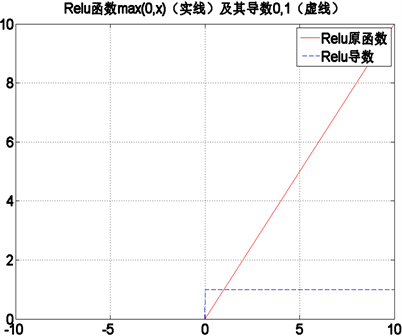Figure 8. Primitive function and derived function of the Relu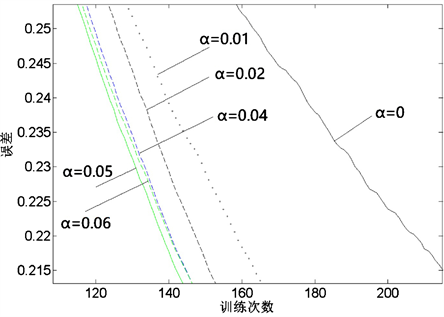Figure 9. Selection of correction coefficient

4. 总结

Research on Deep Learning Algorithm about Handwriting Based on the Convolutional Neural Network[J]. 计算机科学与应用, 2018, 08(11): 1773-1781. https://doi.org/10.12677/CSA.2018.811196

1. 1. 王心宇, 马良, 蔡瑞. 基于卷积神经网络的图像识别[J]. 工程技术研究, 2018(4): 101-102.

2. 2. 朱瑞. 基于卷积神经网络的图像识别算法的研究[D]: [硕士学位论文]. 北京: 北京邮电大学, 2018.

3. 3. 段金宝. 基于深度神经网络的证件图像文本识别方法[D]: [硕士学位论文]. 北京: 北京邮电大学, 2018.

4. 4. 尚泽元. 基于深度区域卷积神经网络图像识别的研究[J]. 中国战略新兴产业, 2017(44): 151-153.

5. 5. 文馗. 基于深度学习的图像识别方法研究与应用[D]: [硕士学位论文]. 武汉: 华中师范大学, 2017.

6. 6. 焦李成, 杨淑媛, 刘芳, 王士刚, 冯志玺. 神经网络七十年: 回顾与展望[J]. 计算机学报, 2016, 39(8): 1697-1716.

7. 7. 陈雪. 神经网络的图像识别技术及方法分析[J]. 通讯世界, 2016(1): 39-40.

8. 8. 战国科. 基于人工神经网络的图像识别方法研究[D]: [硕士学位论文]. 北京: 中国计量科学研究院, 2007.

9. 9. 彭淑敏. 神经网络图像识别技术研究与实现[D]: [硕士学位论文]. 西安: 西安电子科技大学, 2005.